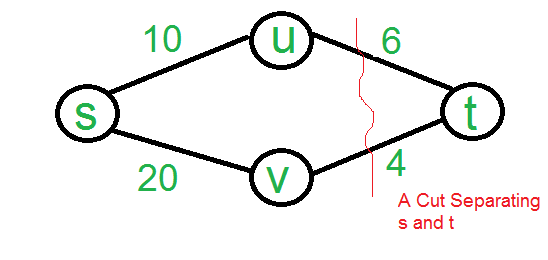GATE | GATE-CS-2005 | Question 83

• Last Updated : 28 Jun, 2021

Let s and t be two vertices in a undirected graph G + (V, E) having distinct positive edge weights. Let [X, Y] be a partition of V such that s ∈ X and t ∈ Y. Consider the edge e having the minimum weight amongst all those edges that have one vertex in X and one vertex in Y

The edge e must definitely belong to:
(A) the minimum weighted spanning tree of G
(B) the weighted shortest path from s to t
(C) each path from s to t
(D) the weighted longest path from s to t

Explanation: The minimum weight edge on any s-t cut is always part of MST. This is called Cut Property.

This is the idea used in Prim’s algorithm. The minimum weight cut edge is always a minimum spanning tree edge.

Why B (the weighted shortest path from s to t) is not an answer?
See below example, edge 4 (lightest in highlighted red cut from s to t) is not part of shortest path.Quiz of this Question

My Personal Notes arrow_drop_up